Like   Tweet   in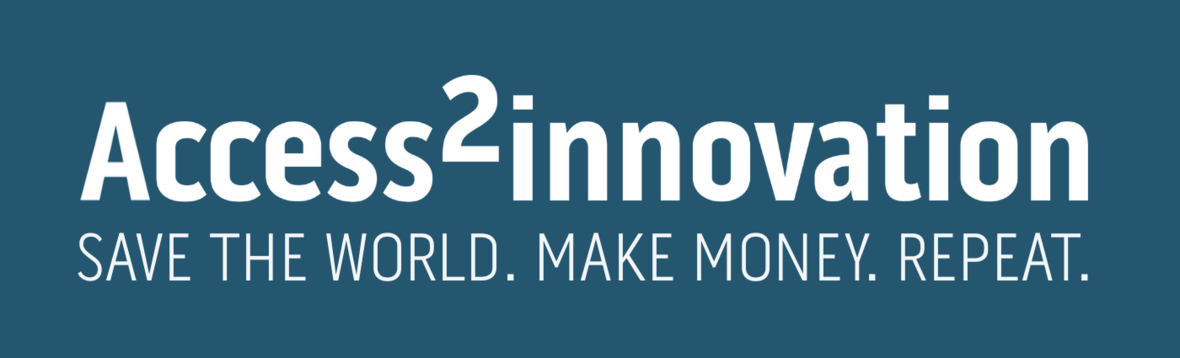## News from Access2innovation September 2023

 table div table+table+table div table{width:100%;padding:0}table div table+table+table div table img{width:96.23%;padding:0;float:none}table div table+table+table div table td{width:100%;padding:0 1.88% 18px}/* styles */# We invite students to join project on business development in Africa

 table div table+table+table+table+table div table{width:100%;padding:0}table div table+table+table+table+table div table img{width:96.23%;padding:0;float:none}table div table+table+table+table+table div table td{width:100%;padding:0 1.88% 18px}/* styles */## Meet our new program manager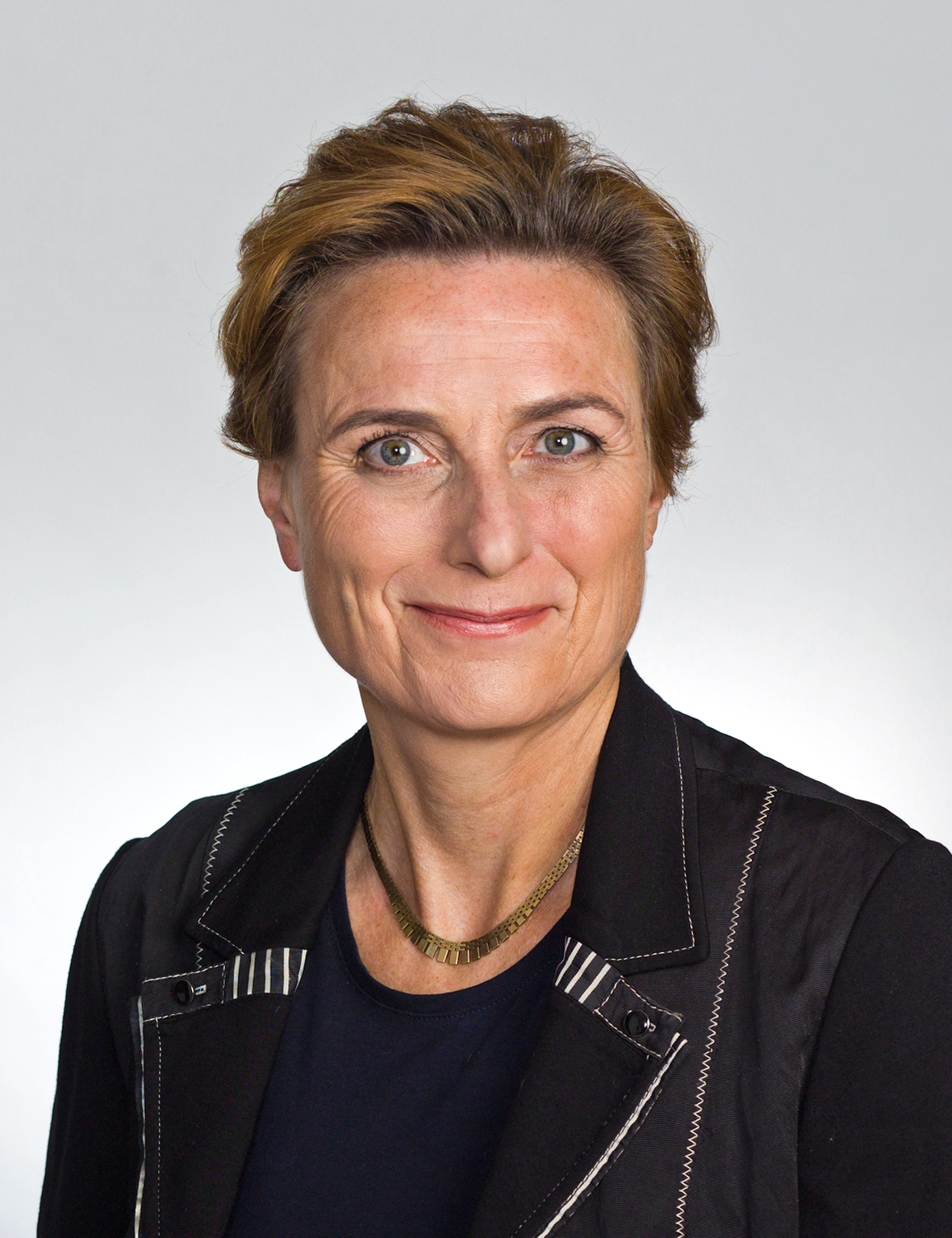/* styles */ A warm welcome to our new program manager, Inge Myrthue. She has more than 20 years of experience working with Sales, business development, and establishing strategic partnerships in a multi-cultural setting in Emerging markets. She has tendered technical projects to governments, the UN, and larger Key Accounts and has experience in the on-site implementation of the activities. Inge specialized in dialogue, negotiation, and building of complex and long-term partnerships with the private and public sectors and NGOs in Africa. “I am really excited to welcome Inge to access2innovation where she will add great value and complementary to the team with her extensive knowledge and experience – especially as we embark on new projects within green transition and energy,” says CEO in Access2innovation, Karin Elisabeth Lind. Click here to read more about Inge Myrthue (in Danish)
 table div table+table+table+table+table+table+table+table+table div table{width:100%;padding:0}table div table+table+table+table+table+table+table+table+table div table img{width:96.23%;padding:0;float:none}table div table+table+table+table+table+table+table+table+table div table td{width:100%;padding:0 1.88% 18px}/* styles */## Next A2i Talk: Securing capital and know-how through active investors and board members

 table.module-10{width:75.47%;padding:0}table div table+table+table+table+table+table+table+table+table+table+table div table{width:75.47%;float:none;margin-left:auto;margin-right:auto;padding:0}table div table+table+table+table+table+table+table+table+table+table+table div table a{border:0 none;text-decoration:none}table div table+table+table+table+table+table+table+table+table+table+table div table img{width:100%!important;border:0 none;text-decoration:none}table div table+table+table+table+table+table+table+table+table+table+table div table td{width:100%;padding:0}/* styles */
 /* styles */ In our next A2i-talk, CEO and founder of Lake Victoria Vanilla in Tanzania, Mette Ravn will take us through her journey of securing investors and competent board members for her company. She will talk about her approach and key considerations in attracting investors and board members that not only contribute to capital, but also adds valuable business insights to the development of the company. All this in the context of developing a sustainable vanilla farm in rural Tanzania. See you at the virtual A2i Talk September 8th, 9.30-10.15 CEST, when you find the us here. Please register and read more here.
 table div table+table+table+table+table+table+table+table+table+table+table+table+table div table{width:100%;padding:0}table div table+table+table+table+table+table+table+table+table+table+table+table+table div table img{width:96.23%;padding:0;float:none}table div table+table+table+table+table+table+table+table+table+table+table+table+table div table td{width:100%;padding:0 1.88% 18px}/* styles */## New members

 /* styles */

This month we say welcome to two new, valuated members of our network:

▪ Caribu
▪ SustaiNet
 ▪ Caribu

 table div table+table+table+table+table+table+table+table+table+table+table+table+table+table+table+table+table+table div table{width:100%;padding:0}table div table+table+table+table+table+table+table+table+table+table+table+table+table+table+table+table+table+table div table img{width:96.23%;padding:0;float:none}table div table+table+table+table+table+table+table+table+table+table+table+table+table+table+table+table+table+table div table td{width:100%;padding:0 1.88% 18px}/* styles */## Sidste chance: Delegationstur til Kenya og Tanzania

 /* styles */ Du kan stadig nå at tilmelde dig vores delegationstur i september til Kenya og Tanzania. Men det haster - sidste tilmelding er i start uge 36 (helst senest 4.9.23). Vi lægger et program, så du møder præcis de myndigheder og potentielle samarbejdspartnere, som du får brug for til realisering af din businessplan. Læs mere her.
 table div table+table+table+table+table+table+table+table+table+table+table+table+table+table+table+table+table+table+table+table+table div table td,table.module-20{width:100%;padding:0}table div table+table+table+table+table+table+table+table+table+table+table+table+table+table+table+table+table+table+table+table+table div table{width:100%;float:none;margin-left:auto;margin-right:auto;padding:0}table div table+table+table+table+table+table+table+table+table+table+table+table+table+table+table+table+table+table+table+table+table div table a{border:0 none;text-decoration:none}table div table+table+table+table+table+table+table+table+table+table+table+table+table+table+table+table+table+table+table+table+table div table img{width:100%!important;border:0 none;text-decoration:none}/* styles */
 table div table+table+table+table+table+table+table+table+table+table+table+table+table+table+table+table+table+table+table+table+table+table div table{width:100%;padding:0}table div table+table+table+table+table+table+table+table+table+table+table+table+table+table+table+table+table+table+table+table+table+table div table img{width:96.23%;padding:0;float:none}table div table+table+table+table+table+table+table+table+table+table+table+table+table+table+table+table+table+table+table+table+table+table div table td{width:100%;padding:0 1.88% 18px}/* styles */## "Thrilled to partner"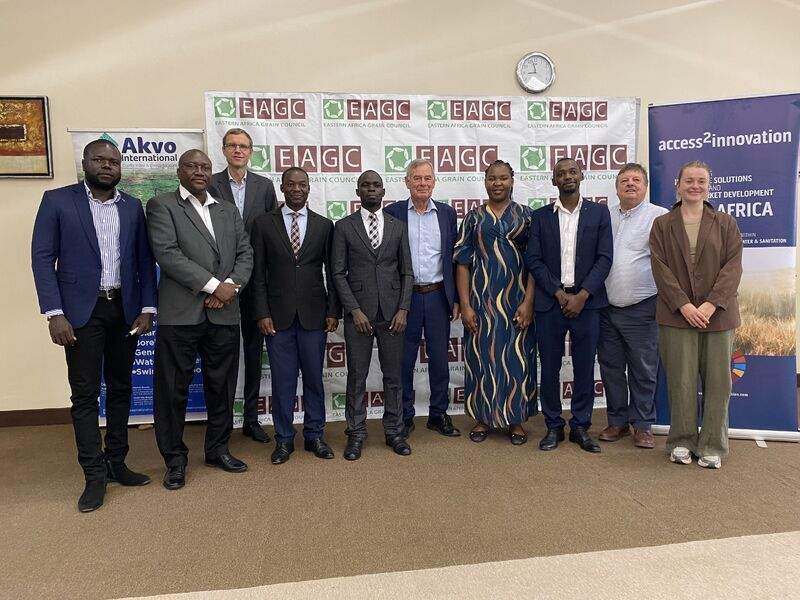The Danish Embassy in Uganda writes about our cooperation:
" ..thrilled to partner with GRUNDFOS, Access2innovation, Akvo, and EAGC. Our joint effort aims at enhancing climate resilience through innovative solar-powered irrigation systems, empower small-holder farmers, and promote sustainable agricultural practices."

 table div table+table+table+table+table+table+table+table+table+table+table+table+table+table+table+table+table+table+table+table+table+table+table+table+table div table{width:100%;padding:0}table div table+table+table+table+table+table+table+table+table+table+table+table+table+table+table+table+table+table+table+table+table+table+table+table+table div table img{width:96.23%;padding:0;float:none}table div table+table+table+table+table+table+table+table+table+table+table+table+table+table+table+table+table+table+table+table+table+table+table+table+table div table td{width:100%;padding:0 1.88% 18px}/* styles */## Are you a student and interested in business in Africa?

 /* styles */ We invite one or two students at master level to conduct a study in collaboration with us, of the business models and strategies that Danish firms have and are using to enter and penetrate African markets. Click here to read more.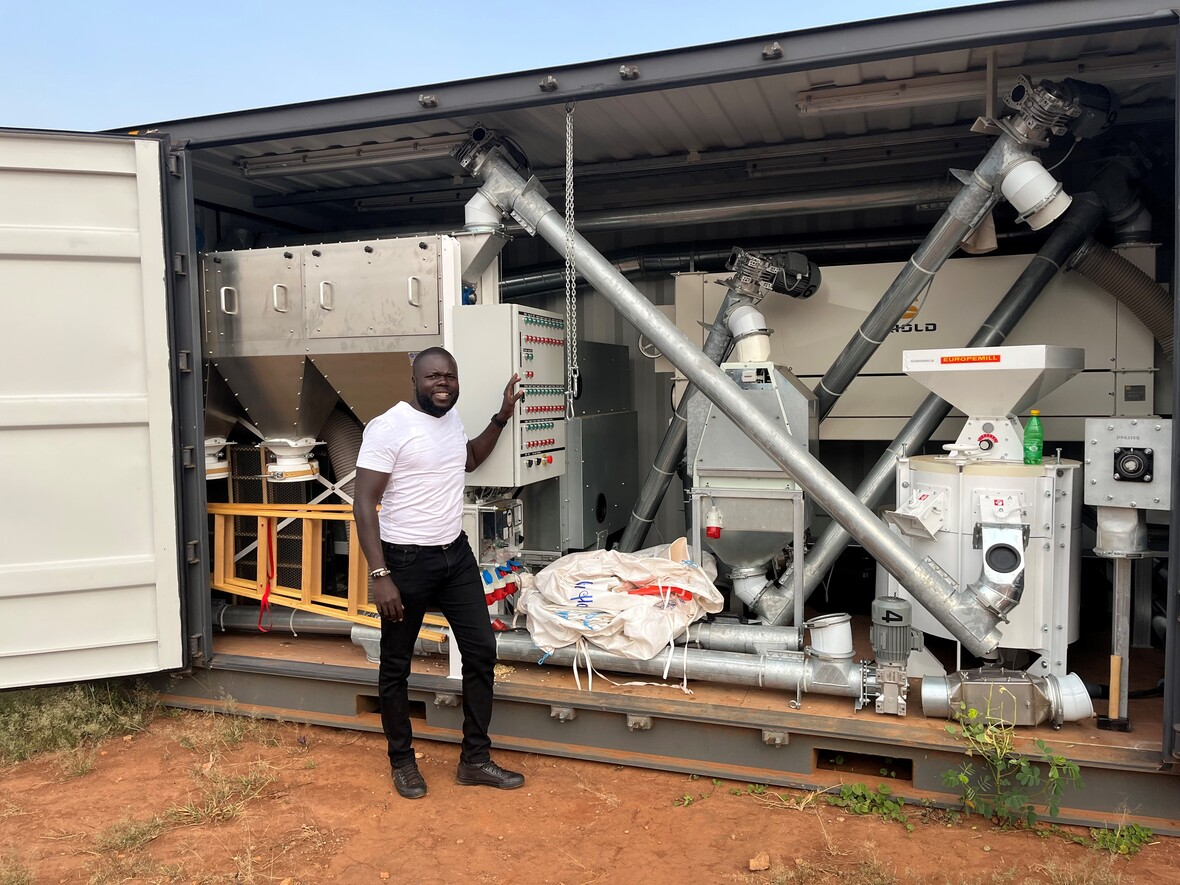Danish tech ready for African grain in our current DMDP-project in Uganda.
 table div table+table+table+table+table+table+table+table+table+table+table+table+table+table+table+table+table+table+table+table+table+table+table+table+table+table+table+table+table div table{width:100%;padding:0}table div table+table+table+table+table+table+table+table+table+table+table+table+table+table+table+table+table+table+table+table+table+table+table+table+table+table+table+table+table div table img{width:96.23%;padding:0;float:none}table div table+table+table+table+table+table+table+table+table+table+table+table+table+table+table+table+table+table+table+table+table+table+table+table+table+table+table+table+table div table td{width:100%;padding:0 1.88% 18px}/* styles */## Input on business development in Afrika

 table div table+table+table+table+table+table+table+table+table+table+table+table+table+table+table+table+table+table+table+table+table+table+table+table+table+table+table+table+table+table+table div table,table.module-30{width:71.7%;float:left;padding:0}table div table+table+table+table+table+table+table+table+table+table+table+table+table+table+table+table+table+table+table+table+table+table+table+table+table+table+table+table+table+table+table div table a{border:0 none;text-decoration:none}table div table+table+table+table+table+table+table+table+table+table+table+table+table+table+table+table+table+table+table+table+table+table+table+table+table+table+table+table+table+table+table div table img{width:100%!important;border:0 none;text-decoration:none}table div table+table+table+table+table+table+table+table+table+table+table+table+table+table+table+table+table+table+table+table+table+table+table+table+table+table+table+table+table+table+table div table td{width:100%;padding:0 20px 20px 0}/* styles */ You can always get free input about business in Africa from our A2i Talk videos. Remember, that you can also get one-on-one sparring and advice if you a strategic member of Access2innovation. Reach out to one of our colleagues directly. Find us here.
 table div table+table+table+table+table+table+table+table+table+table+table+table+table+table+table+table+table+table+table+table+table+table+table+table+table+table+table+table+table+table+table+table div table{width:100%;padding:0}table div table+table+table+table+table+table+table+table+table+table+table+table+table+table+table+table+table+table+table+table+table+table+table+table+table+table+table+table+table+table+table+table div table img{width:96.23%;padding:0;float:none}table div table+table+table+table+table+table+table+table+table+table+table+table+table+table+table+table+table+table+table+table+table+table+table+table+table+table+table+table+table+table+table+table div table td{width:100%;padding:0 1.88% 18px}/* styles */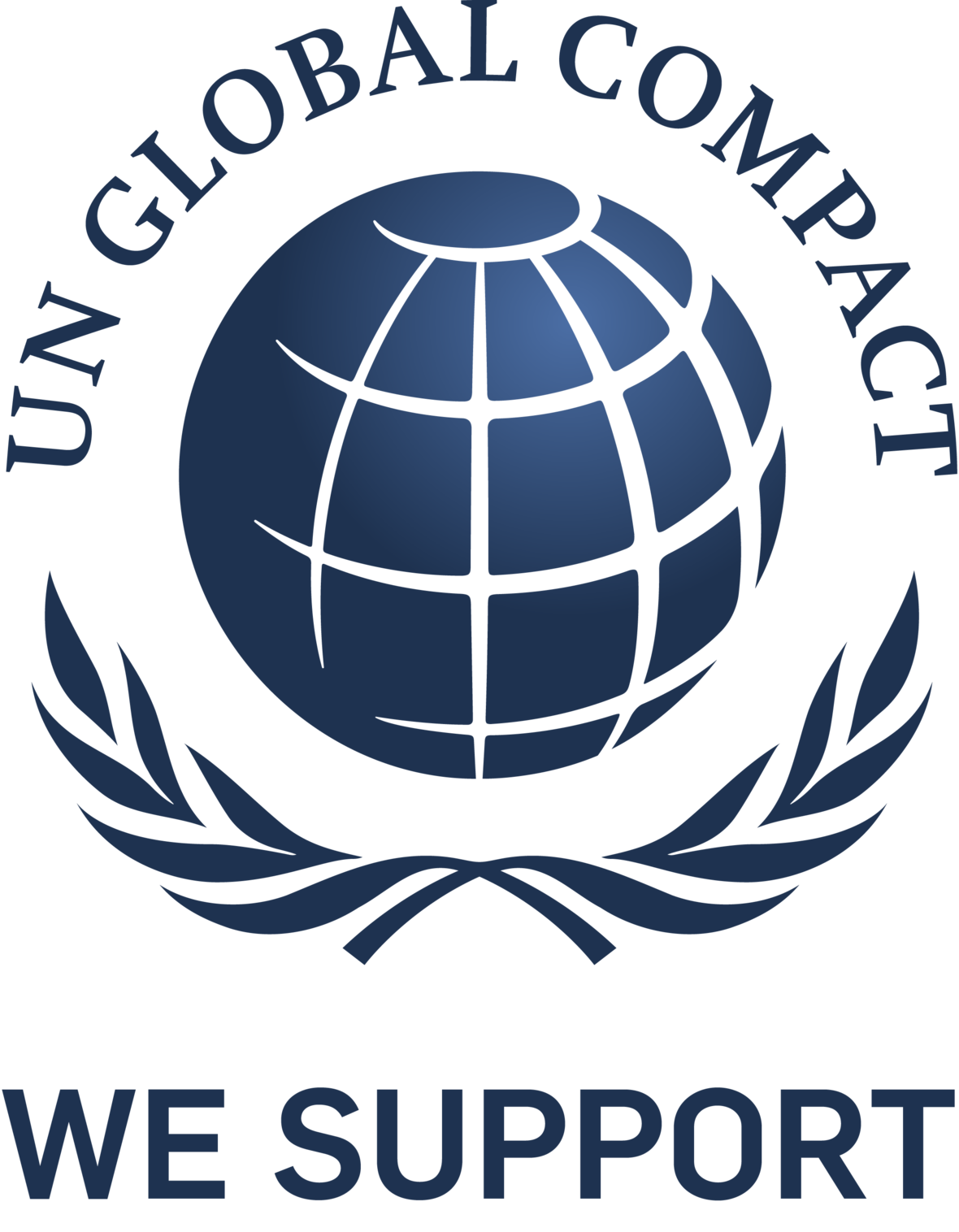table div table+table+table+table+table+table+table+table+table+table+table+table+table+table+table+table+table+table+table+table+table+table+table+table+table+table+table+table+table+table+table+table+table+table div table{width:100%;padding:0}table div table+table+table+table+table+table+table+table+table+table+table+table+table+table+table+table+table+table+table+table+table+table+table+table+table+table+table+table+table+table+table+table+table+table div table img{width:96.23%;padding:0;float:none}table div table+table+table+table+table+table+table+table+table+table+table+table+table+table+table+table+table+table+table+table+table+table+table+table+table+table+table+table+table+table+table+table+table+table div table td{width:100%;padding:0 1.88% 18px}/* styles *//* styles */ Access2innovation er den danske platform, hvorfra danske virksomheder i samarbejde med NGO’er, investorer, vidensinstitutioner og myndigheder udvikler og skalerer innovative og bæredygtige, kommercielle løsninger til de afrikanske vækstmarkeder. Send gerne videre! Tilmeld dig nyhedsbrevet her, hvis du ikke allerede er tilmeldt.
 table div table+table+table+table+table+table+table+table+table+table+table+table+table+table+table+table+table+table+table+table+table+table+table+table+table+table+table+table+table+table+table+table+table+table+table+table div table{width:100%;padding:0}table div table+table+table+table+table+table+table+table+table+table+table+table+table+table+table+table+table+table+table+table+table+table+table+table+table+table+table+table+table+table+table+table+table+table+table+table div table img{width:96.23%;padding:0;float:none}table div table+table+table+table+table+table+table+table+table+table+table+table+table+table+table+table+table+table+table+table+table+table+table+table+table+table+table+table+table+table+table+table+table+table+table+table div table td{width:100%;padding:0 1.88% 18px}/* styles */